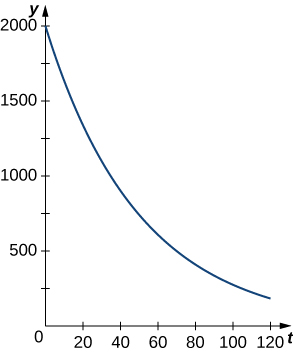## Exponential Decay Model

### Learning Outcomes

• Use the exponential decay model in applications, including radioactive decay and Newton’s law of cooling
• Explain the concept of half-life

Exponential functions can also be used to model populations that shrink (from disease, for example), or chemical compounds that break down over time. We say that such systems exhibit exponential decay, rather than exponential growth. The model is nearly the same, except there is a negative sign in the exponent. Thus, for some positive constant $k,$ we have $y={y}_{0}{e}^{\text{−}kt}.$

As with exponential growth, there is a differential equation associated with exponential decay. We have

${y}^{\prime }=\text{−}k{y}_{0}{e}^{\text{−}kt}=\text{−}ky.$

### Exponential Decay Model

Systems that exhibit exponential decay behave according to the model

$y={y}_{0}{e}^{\text{−}kt},$

where ${y}_{0}$ represents the initial state of the system and $k>0$ is a constant, called the decay constant.

The following figure shows a graph of a representative exponential decay function.Figure 2. An example of exponential decay.

Let’s look at a physical application of exponential decay. Newton’s law of cooling says that an object cools at a rate proportional to the difference between the temperature of the object and the temperature of the surroundings. In other words, if $T$ represents the temperature of the object and ${T}_{a}$ represents the ambient temperature in a room, then

${T}^{\prime }=\text{−}k(T-{T}_{a}).$

Note that this is not quite the right model for exponential decay. We want the derivative to be proportional to the function, and this expression has the additional ${T}_{a}$ term. Fortunately, we can make a change of variables that resolves this issue. Let $y(t)=T(t)-{T}_{a}.$ Then ${y}^{\prime }(t)={T}^{\prime }(t)-0={T}^{\prime }(t),$ and our equation becomes

${y}^{\prime }=\text{−}ky.$

From our previous work, we know this relationship between $y$ and its derivative leads to exponential decay. Thus,

$y={y}_{0}{e}^{\text{−}kt},$

and we see that

$\begin{array}{ccc}\hfill T-{T}_{a}& =\hfill & ({T}_{0}-{T}_{a}){e}^{\text{−}kt}\hfill \\ \hfill T& =\hfill & ({T}_{0}-{T}_{a}){e}^{\text{−}kt}+{T}_{a}\hfill \end{array}$

where ${T}_{0}$ represents the initial temperature. Let’s apply this formula in the following example.

### Example: Newton’s Law of Cooling

According to experienced baristas, the optimal temperature to serve coffee is between $155\text{°}\text{F}$ and $175\text{°}\text{F}.$ Suppose coffee is poured at a temperature of $200\text{°}\text{F},$ and after 2 minutes in a $70\text{°}\text{F}$ room it has cooled to $180\text{°}\text{F}.$ When is the coffee first cool enough to serve? When is the coffee too cold to serve? Round answers to the nearest half minute.

### Try It

Suppose the room is warmer $(75\text{°}\text{F})$ and, after 2 minutes, the coffee has cooled only to $185\text{°}\text{F}.$ When is the coffee first cool enough to serve? When is the coffee be too cold to serve? Round answers to the nearest half minute.

Watch the following video to see the worked solution to the above Try It.

For closed captioning, open the video on its original page by clicking the Youtube logo in the lower right-hand corner of the video display. In YouTube, the video will begin at the same starting point as this clip, but will continue playing until the very end.

### Try It

Just as systems exhibiting exponential growth have a constant doubling time, systems exhibiting exponential decay have a constant half-life. To calculate the half-life, we want to know when the quantity reaches half its original size. Therefore, we have

$\begin{array}{ccc}\hfill \frac{{y}_{0}}{2}& =\hfill & {y}_{0}{e}^{\text{−}kt}\hfill \\ \hfill \frac{1}{2}& =\hfill & {e}^{\text{−}kt}\hfill \\ \hfill -\text{ln}2& =\hfill & \text{−}kt\hfill \\ \hfill t& =\hfill & \frac{\text{ln}2}{k}.\hfill \end{array}$

Note: This is the same expression we came up with for doubling time.

### Definition

If a quantity decays exponentially, the half-life is the amount of time it takes the quantity to be reduced by half. It is given by

$\text{Half-life}=\frac{\text{ln}2}{k}$

One of the most common applications of an exponential decay model is carbon dating. $\text{Carbon-}14$ decays (emits a radioactive particle) at a regular and consistent exponential rate. Therefore, if we know how much carbon was originally present in an object and how much carbon remains, we can determine the age of the object. The half-life of $\text{carbon-}14$ is approximately 5730 years—meaning, after that many years, half the material has converted from the original $\text{carbon-}14$ to the new nonradioactive $\text{nitrogen-}14.$ If we have 100 g $\text{carbon-}14$ today, how much is left in 50 years? If an artifact that originally contained 100 g of carbon now contains 10 g of carbon, how old is it? Round the answer to the nearest hundred years.
If we have $100$ g of $\text{carbon-}14,$ how much is left after $t$ years? If an artifact that originally contained $100$ g of carbon now contains $20g$ of carbon, how old is it? Round the answer to the nearest hundred years.